1. /
2. CBSE
3. /
4. Class 06
5. /
6. Mathematics
7. /
8. NCERT Solutions for Class...

# NCERT Solutions for Class 6 Maths Exercise 13.1

NCERT solutions for Maths Symmetry## NCERT Solutions for Class 6 Maths Symmetry

###### Question 2.For the given figure, which one is the mirror line, {tex}{l_1}{/tex} or {tex}{l_2}?{/tex}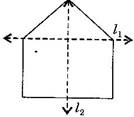Answer: {tex}{l_2}{/tex} is the mirror line as both sides of the lines are symmetric.

NCERT Solutions for Class 6 Maths Exercise 13.1

###### Question 3.Identify the shapes given below. Check whether they are symmetric or not. Draw the line of symmetry as well.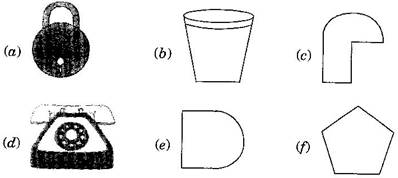(a) Symmetric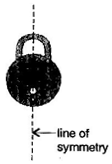(b) Symmetric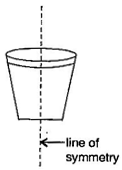(c) Not symmetric

(d) Symmetric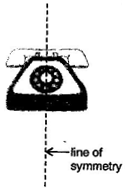(e) Symmetric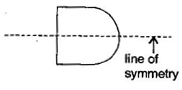(f)Symmetric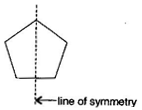NCERT Solutions for Class 6 Maths Exercise 13.1

###### Question 4.Copy the following on a square paper. A square paper is what you would have used in your arithmetic notebook in earlier classes. Then complete them such that the dotted line is the line of symmetry.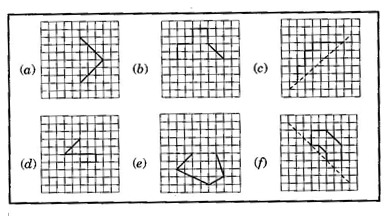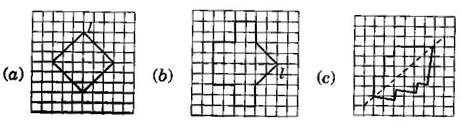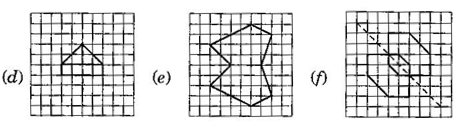###### Question 5.In the figure, {tex}l{/tex} is the line of symmetry. Complete the diagram to make it symmetric.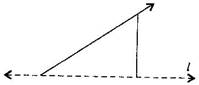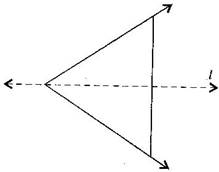NCERT Solutions for Class 6 Maths Exercise 13.1

###### Question 6.In the figure, {tex}l{/tex} is the line of symmetry. Draw the image of the triangle and complete the diagram, so that it becomes symmetric.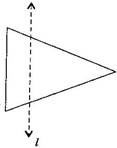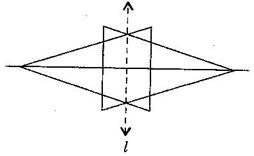## NCERT Solutions for Class 6 Maths Exercise 13.1

NCERT Solutions Class 6 Maths PDF (Download) Free from myCBSEguide app and myCBSEguide website. Ncert solution class 6 Maths includes text book solutions from Class 6 Maths Book . NCERT Solutions for CBSE Class 6 Maths have total 14 chapters. 6 Maths NCERT Solutions in PDF for free Download on our website. Ncert Maths class 6 solutions PDF and Maths ncert class 6 PDF solutions with latest modifications and as per the latest CBSE syllabus are only available in myCBSEguide.

## CBSE app for Students

To download NCERT Solutions for Class 6 Maths, Social Science Computer Science, Home Science, Hindi English, Maths Science do check myCBSEguide app or website. myCBSEguide provides sample papers with solution, test papers for chapter-wise practice, NCERT solutions, NCERT Exemplar solutions, quick revision notes for ready reference, CBSE guess papers and CBSE important question papers. Sample Paper all are made available through the best app for CBSE students and myCBSEguide website.### Test Generator

Create question paper PDF and online tests with your own name & logo in minutes.### myCBSEguide

Question Bank, Mock Tests, Exam Papers, NCERT Solutions, Sample Papers, Notes

### 2 thoughts on “NCERT Solutions for Class 6 Maths Exercise 13.1”

1. Identify the symetrical instrument from your mathematical instrument box

2. dama dam mast calander